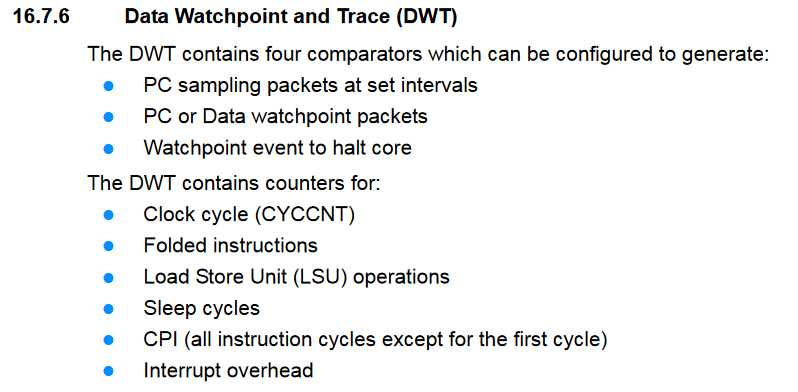## Calculating the time taken for a task in SAMV71

4 posts / 0 new
Author
Message

Hello,

I need to calculate the time taken for 300 loops of my code. In arduino I used to use millis( ). Is there an equivalent of millis() in ASK for SAMV71?I came across this page in ASF http://asf.atmel.com/docs/latest/samg/html/time__tick__sam_8c.html#ad8f342ce7143e60a1a3f70234e446644 but when I tried to include time_tick.h I get an error saying no such file or directory. Here is my code for calculating the total loops time where i is the loop counter. This code does not work as the library is not found.

```if(i==0)
{
tick_start = time_tick_get();
}

//algo runs

if(i==299)
{
tick_end = time_tick_get();
printf("Time %d \n",tick_end-tick_start);
}```

`time_tick_get is not a library, it's just part of the SD card raw access example. If you create that example project you can copy the files (the .h and .c) and add them to your project.`

/Lars